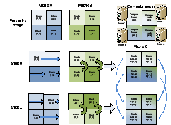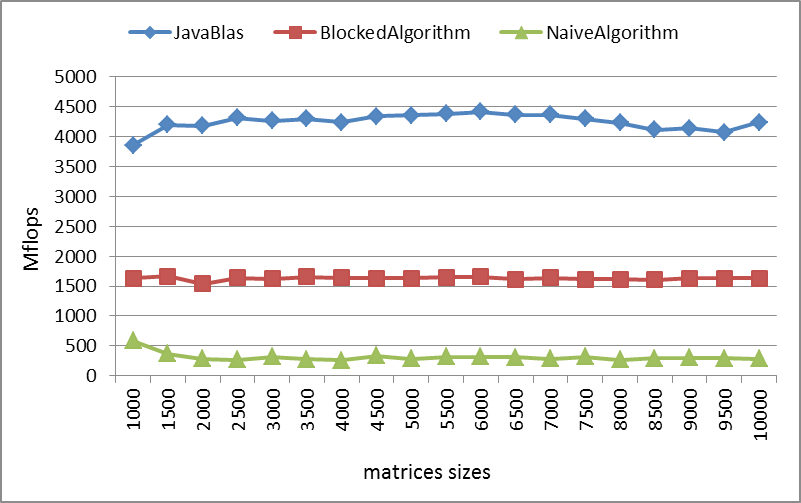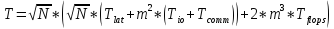# Statement of Problem

 Date 09.06.2018 Size 57.46 Kb. #53773
Modeling Communication Cost for Matrix Multiply in Tree Network Cluster

Hui Li: lihui@indiana.edu

Xiaoyang Chen: xc7@imail.iu.edu

## Statement of Problem

Computers are rational and for most structured parallel applications, it is usually possible to model performance once you know the problem model, machine model, and communication model. The matrix multiply is an important kernel in some scientific problems. It is one of the most well studied algorithms in high performance computing. Specifically, we will study the square full matrix multiply in order to simplify the problem model. In addition, we assume the machine with a fast CPU and very large memory space. And we just consider the machine as a processor with the processing speed of Tflops. Further, we assume the matrix multiply will run in a tree network cluster with the speed of Tcomm, which are the most cases happens in many dedicated cluster. The goal of this study is to model Tcomm/Tflops, the communication overhead per double float point operations, for matrix multiply in dedicated cluster. And the difficulty of work is to model the communication overhead of matrix multiply over the tree network topology.

## Survey

There are several parallel dense matrix multiplication algorithms of the form C=a*A*B+b*C on a mesh of processes. These algorithms can be coherently classified into three categories according to the communication patterns. As no single algorithm always achieves the best performance for multiplying matrices with different sizes on arbitrary process grids, some poly-algorithms is proposed to address this dilemma . Further, there exist some parallel libraries software that provides set of coherent parallel services for linear algebra programs. One advantage of building such parallel libraries is that they may fully exploit the parallelism of a specific problem and effectively utilize the underlying parallel systems, while providing a portable interface specification. A potential disadvantage is that hand-coded software, tuned to the specific processor, segment of network topology, operating system or compiler release will, though at great development cost, outperform portable libraries. Sample libraries include the Basic Linear Algebra Subprogram (BLAS), the Linear Algebra PACKage (LAPACK), and Scalable LAPACK (ScalAPACK).

## Architecture DesignFigure 1. Work flow of Fox Algorithm on Mesh of 2*2 Nodes

## Implementation timeline

1. Survey: milestone1

2. Proposal (Oct, 31)

2. Matrix Multiply in Shared Memory Parallelism Model

1. Port CBlas and JBlas into Matrix Multiply code

3. Performance study

3. Matrix Multiply in Distributed Memory Parallelism Model

1. Port CBlas and JBlas into OpenMPI, Twister, DryadLINQ

2. ScalaPACK

3. Performance analysis

4. Report

## Validation methods

1. Performance Analysis:

1. Absolute performance metric: Mflops per second

2. Relative performance metric: Parallel efficiencyFigure 2. Absolute performance of matrix multiply

1. Timing model :

1. Timing model to analyze Tcomm/Tflops, the communication overhead per double float point calculation

2. Compare predicated job turnaround and the real job turnaround time.